# Understanding AD / DA converters

Generally, an analog-digital converter is an electronic device that converts an analog voltage input to a digital number. The digital output can use different coding schemes, such as binary, although some non-electrical or partially electrical devices can be considered as analog-digital converters.

The resolution of a converter indicates the number of discrete values ​​it can produce over a range of voltage values. It is usually expressed in bits.
For example, a converter that encodes an analog input of 1 to 256 discrete values ​​(0..255) has a resolution of 8 bits: that is, 2 raised to 8. The resolution can also be defined electrically and expressed in volts.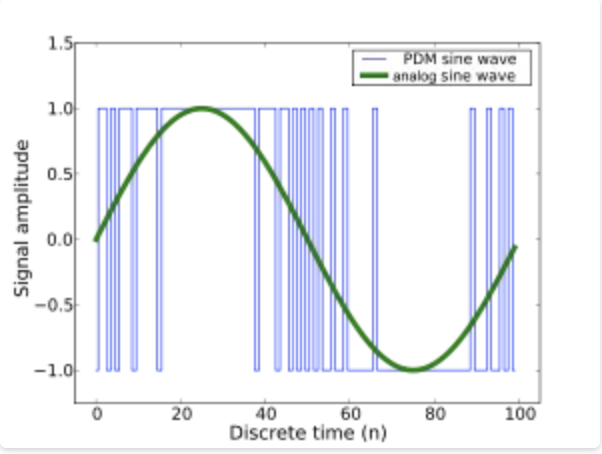### ANALOG SYSTEM AND DIGITAL SYSTEM

Electronic circuits can be divided into two broad categories: digital and analog. Digital electronics use magnitudes with discrete values, while analog electronics use magnitudes with continuous values.

A digital system is any device intended for the generation, transmission, processing or storage of digital signals. Thus, the digital system is a combination of devices designed to manipulate physical quantities or information that are represented in digital form; that is, they can only take discrete values.

For the implementation of digital circuits, logic gates (AND, OR and NOT) and transistors are used. These doors follow the behavior of some functions.

A system is analog when the magnitudes of the signal are represented by continuous variables, this is analogous to the magnitudes that give rise to the generation of this signal. An analog system contains devices that manipulate physical quantities represented in analog form. In a system of this type, quantities vary over a continuous range of values.
Thus, an analog magnitude is one that takes continuous values. A digital quantity is one that takes a set of discrete values.

An analog signal is a voltage or current that varies smoothly and continuously. A sine wave is a single frequency analog signal. The voltages of voice and video are analog signals that vary according to the sound or variations of the light that corresponds to the information being transmitted.

Digital signals, in contrast to analog signals, do not vary continuously but change in steps or in discrete increments.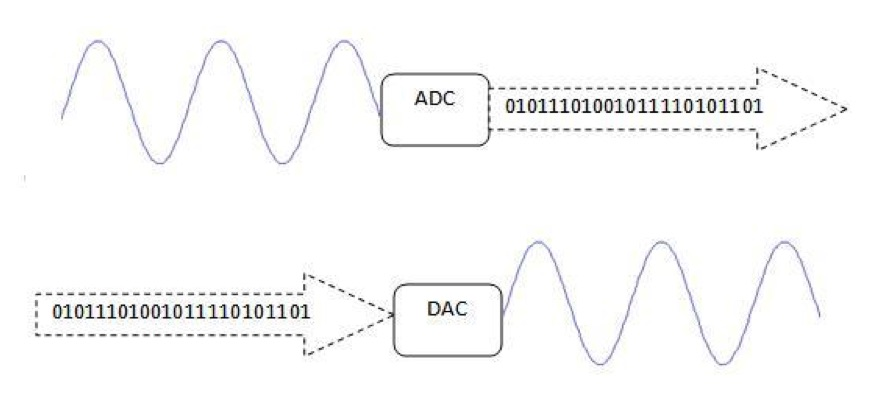• Reproducibility of results – Proof
• Ease of design –
• Flexibility and functionality
• Programmability
• Speed
• Economy
• Constant technological progress

The method of digital signal processing also enables the implementation of more sophisticated signal processing algorithms. It is usually very difficult to perform precise mathematical operations on signals in analog format, but these same operations can be carried out routinely on a digital computer using the software.

The ADC converter (Analog-to-Digital Converter – Digital Analog Converter) has to carry out the following processes:

1.- Sampling of the analog signal.
2.- Quantization of the signal itself
3.- Coding of the result of the quantization, in binary code.

###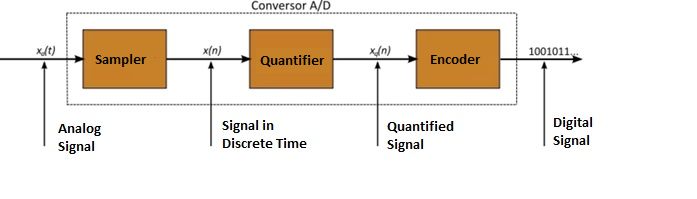The sampling of an analog signal

The graphic representation of half positive cycle (+), corresponding to an analog electrical sound signal, with its corresponding harmonics. As can be seen, the voltage or voltage variation values ​​in this sinusoid can vary on a scale.

To convert an analog signal into digital, the first step is to perform a sampling (sampling) of it, or what is the same, take different samples of voltages or voltages at different points of the sine wave. The frequency at which the sampling is done is called ratio, rate or also sampling frequency and is measured in kilohertz (kHz). In the case of digital audio recording, the greater the number of samples taken, the higher the quality and fidelity of the resulting digital signal.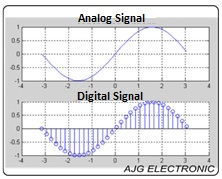During the sampling process, numerical values ​​equivalent to the voltage or voltage existing in different points of the sinusoid are assigned, with the purpose of carrying out the quantization process.

The most commonly used sampling rates or frequencies for digital audio are the following:

• 24,000 samples per second (24 kHz)
• 30,000 samples per second (30 kHz)
• 44 100 samples per second (44.1 kHz) (CD quality)
• 48,000 samples per second (48 kHz)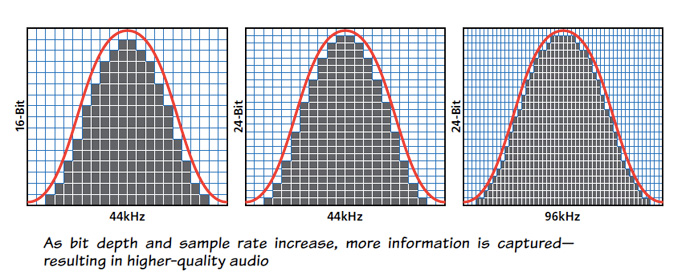To sample an analog electrical signal and then convert it to digital, the first step is to take discrete voltage or voltage values ​​at regular intervals at different points on the sine wave.

Therefore, a signal whose sampling is performed at 24 kHz, will have less quality and fidelity than another made at 48 kHz. However, the greater the number of samples taken, the greater the bandwidth needed to transmit a digital signal, also requiring a much larger space to store it.

### Quantization of the analog signal

The quantization represents the sampling component of the variations of values ​​of voltages or voltages taken in different points of the sine wave, which allows to measure them and assign them their corresponding values ​​in the decimal number system, before converting those values ​​into a binary numerical system.

###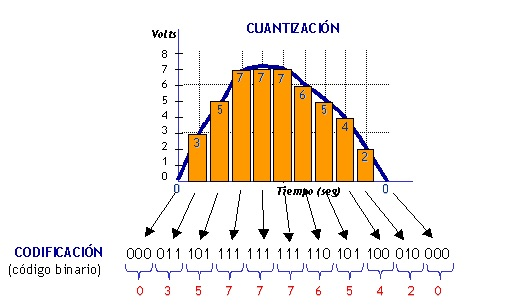Coding of the signal in binary code

After the quantization has been carried out, the values ​​of the voltage taps are represented numerically by previously established codes and standards. The most common is to encode the digital signal in binary code.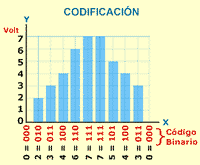An example of an analog electronic system is the speaker, which is used to amplify the sound so that it is heard by a large audience. Sound waves that are analog at their source are captured by a microphone and converted into a small analog voltage variation called an audio signal. This voltage varies continuously as the volume and frequency of the sound change and is applied to the input of a linear amplifier.
The output of the amplifier, which is the amplified input voltage, is fed into the loudspeaker. It converts, again, the amplified audio signal into sound waves with a much higher volume than the original sound picked up by the microphone.

There are systems that use digital and analog methods, one of them is the compact disc player (CD). Music in digital form is stored on the CD. An optical laser diode system reads the digital data from the disk when it rotates and transfers them to the digital-to-analog converter (DAC, digital-to-analog converter). The DAC transforms the digital data into an analog signal that is the electrical reproduction of the original music. This signal is amplified and sent to the speaker. When the music was recorded on the CD, a process was used which, essentially, was the inverse of the one described, and which uses an analog-to-digital converter (ADC).

Browse All SoundGirls Contributors